Example 1: Martha added four and one-fifth packages of soil to her garden on Monday and three and two-fifths packages of soil on Friday. How many packages of soil did she add in all?The fractions have like denominators. We will add the whole numbers and add the fractions separately.

Solution:In example 1,  we arranged the work vertically to make it easier to add the mixed numbers.

To add mixed numbers, add the whole numbers and add the fractions separately: (whole + whole) + (fraction + fraction)

Let's look at some more examples.

Example 2: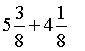Analysis: The fractions have like denominators. Add the whole numbers and add the fractions separately.

Solution: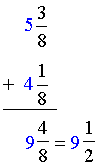In example 2, it was necessary to simplify the result.

Example 3: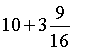Analysis: We are adding a whole number and a mixed number. Think of ten as "ten and zero-sixteenths."

Solution:Example 4: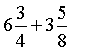Analysis: The fractions have unlike denominators. We will write equivalent fractions using the LCD, 8.

Solution: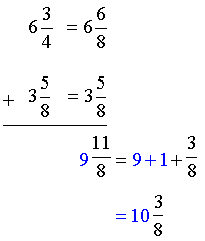Example 5: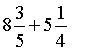Analysis: The fractions have unlike denominators. We will write equivalent fractions using the LCD, 20.

Solution: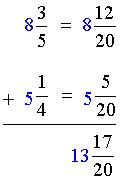Example 6: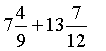Analysis: The fractions have unlike denominators. We will write equivalent fractions using the LCD, 36.

Solution:Example 7: An ice cream vendor sold eighteen and five-sixths liters of ice cream on Friday, and nineteen and one-half liters of ice cream on Saturday. How many liters did he sell in all?

Analysis: The fractions have unlike denominators. We will write equivalent fractions using the LCD, 6.

Solution: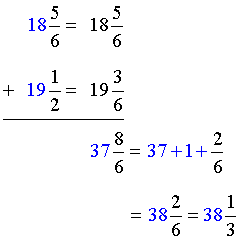1. Examine the fractional part of each mixed number to determine whether the denominators are like or unlike.
2. If the denominators are unlike, use the LCD to rewrite them as equivalent fractions.
3. Add the whole numbers and add the fractions separately: (whole + whole) + (fraction + fraction)
4. Simplify the result, if necessary.

### Exercises

Directions: Add the mixed numbers in each exercise below. Be sure to simplify your result, if necessary. Click once in an ANSWER BOX and type in your answer; then click ENTER. After you click ENTER, a message will appear in the RESULTS BOX to indicate whether your answer is correct or incorrect. To start over, click CLEAR.

Note: To write the mixed number four and two-thirds, enter 4, a space, and then 2/3 into the form.

 1.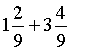ANSWER BOX:   RESULTS BOX:
 2.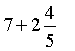ANSWER BOX:   RESULTS BOX:
 3.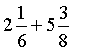ANSWER BOX:   RESULTS BOX:
 4.ANSWER BOX:   RESULTS BOX:
 5. In one week, the Glosser family drank one and seven-twelfths cartons of regular milk and four and one-twelfth cartons of soy milk. How much milk did they drink in all? ANSWER BOX:   cartons   RESULTS BOX:
 Lessons on Adding and Subtracting Fractions and Mixed Numbers 1. Adding Fractions with Like Denominators 2. Subtracting Fractions with Like Denominators 3. Adding and Subtracting Fractions with Unlike Denominators 4. Adding Mixed Numbers 5. Subtracting Mixed Numbers 6. Solving Word Problems 7. Practice Exercises 8. Challenge Exercises 9. Solutions